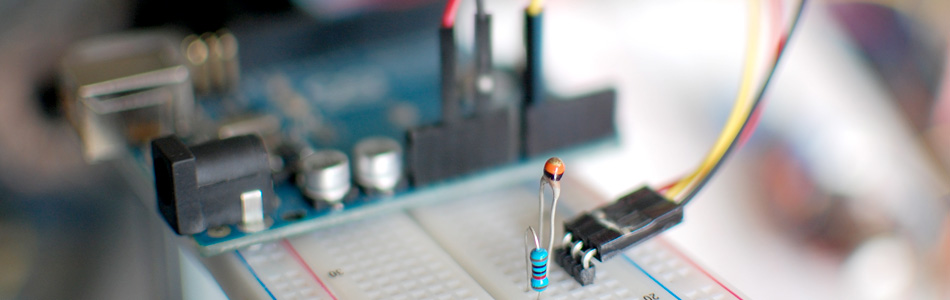A Thermistor is a thermal-resistor. It's just a simple device that changes it's resistance based on temperature. If the LRD/Photoresistor is day of of arduino class. The thermistor should be day 1.01. (Can I do that?).

If you need precise temperature readings, this is not the part for you. Check out the DS18B20, TMP102, or MLX90614

Thermistors are not that precise or anything, so you wont be able to tell the temperature with it, but if you need to know when the temperature has changed, this will work for you. And on the plus side, they are crazy cheap considering the alternatives, incredibly simple to hookup, and have some of the easiest code ever. You can find these pretty easily at most hobby electronics shops, or just add some to your next sparkfun order.

Hooking it up, and why

The thermistor changes its resistance with temperature so we can measure that change using one of the Arduino's analog pins. But to do that we need a fixed resistor (not changing) that we can use for that comparison (We are using a 10K resistor). This is called a voltage divider and divides the 5v between the thermistor and the resistor.

The analog read on your arduino is basically a voltage meter. at 5V (its max) it would read 1023, and at 0v it read 0. So we can measure how much voltage is on the thermistor using the analogRead and we have our reading.

The amount of that 5V that each part gets is proportional to its resistance. So if the the thermistor and the resistor have the same resistance, the 5V is split evenly (2.5V) to each part. (analog reading of 512)

But if the thermistor is really hot and is reading only 1K of resistance, the 10K resistor is going to soak up 10 times as much of that 5V. So the thermistor would only get .45V. (analog reading of 92)

And if it is in the refrigerator, the thermistor may be 40K or resistance, so the thermistor will soak up 4 times as much of that 5V as the 10K resistor. So the thermistor would get 4V. (analog reading of 819)

Code

The arduino code for this just could not be easier. We are adding some serial prints and delays to it just so you can easily see the readings, but they dont need to be there if you dont need them.

int thermistorPin = A0; //analog pin 0

void setup(){
Serial.begin(9600);
}

void loop(){